﻿ Common Core Standards for Math - KindergartenAdaptive Worksheets

Helping Every Child to Succeed

toll-free (888) 777-0876
fax (888) 777-0875

## Understand the Place Value System

5.NBT.A.1
Recognize that in a multi-digit whole number, a digit in one place represents ten times what it represents in the place to its right and 1/10 of what it of what it represents in its place to the left.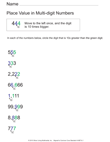Place Value in Multi-Digit Numbers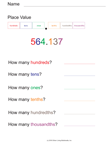Place Value Including Tenths and Hundredths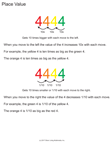Place Value - Compare Digits

5.NBT.A.2

Explain patterns in the number of zeros of the product when multiplying a number by powers of 10, and explain patterns in the placement of the decimal point when a decimal is multiplied or divided by a power of 10. Use whole-number exponents to denote powers of 10.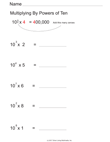Multiply A Whole Number By Powers of Ten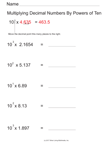Multiply Decimal Numbers By Powers of Ten

5.NBT.A.3
Read, write, and compare decimals to thousandths.

5.NBT.A.3.A
Read and write decimals to thousandths using base-ten numerals, number names, and expanded form, e.g., 347.392 = 3 × 100 + 4 × 10 + 7 × 1 + 3 × (1/10) + 9 × (1/100) + 2 × (1/1000).Decimal Numbers Expanded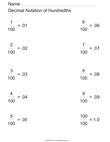Decimal Notation of Hundredths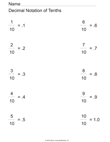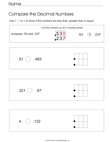Compare Decimal Numbers (With Hints)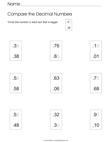Compare Decimal Numbers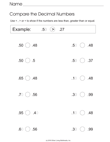Compare Decimal Numbers to Hundredths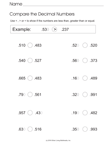Compare Decimal Numbers to Thousandths

5.NBT.A.3.B
Compare two decimals to thousandths based on meanings of the digits in each place, using >, =, and < symbols to record the results of comparisons.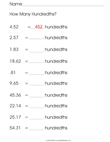How Many Hundredths?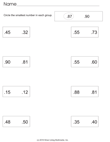Which Decimal Number is the Smallest?

5.NBT.A.4
Use place value understanding to round decimals to any place.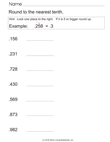Round Decimal Numbers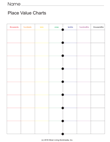Place Value Chart with Decimals

## 5.NBT.B.5 Fluently multiply multi-digit whole numbers using the standard algorithm.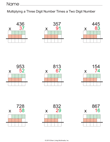Multiply Multiple Digits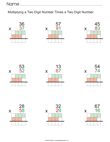Multiply More Multiple Digits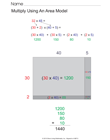Multiply Using an Area Model

## 5.NBT.B.6

Find whole-number quotients of whole numbers with up to four-digit dividends and two-digit divisors, using strategies based on place value, the properties of operations, and/or the relationship between multiplication and division. Illustrate and explain the calculation by using equations, rectangular arrays, and/or area models.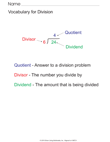Vocabulary for Long Division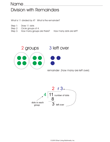Division With Remainders (Visual Prompts)Division With Remainders (Visual Prompts)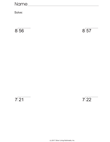Long Division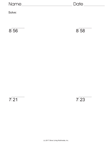More Long Division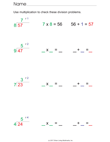Check The Long Division Answers

5.NBT.B.7
Add, subtract, multiply, and divide decimals to hundredths, using concrete models or drawings and strategies based on place value, properties of operations, and/or the relationship between addition and subtraction; relate the strategy to a written method and explain the reasoning used.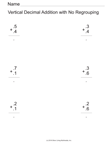Decimal Addition - 1 digit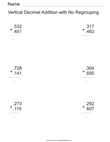Decimal Addition - No Regrouping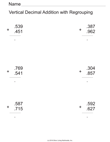Decimal Addition - 3 digits with Regrouping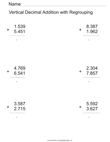Decimal Addition - 4 digits with Regrouping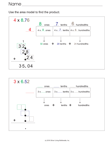Decimal Products - Area Model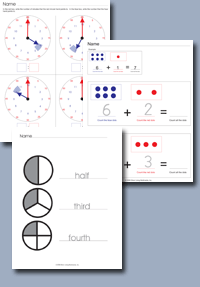Click on any of the standards on the left to create custom worksheets or to print premade materials.